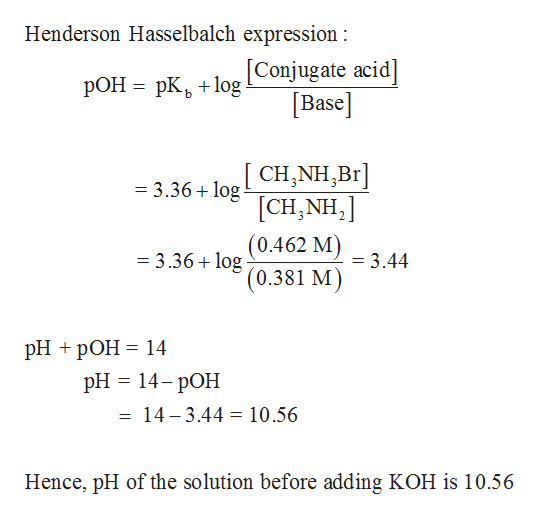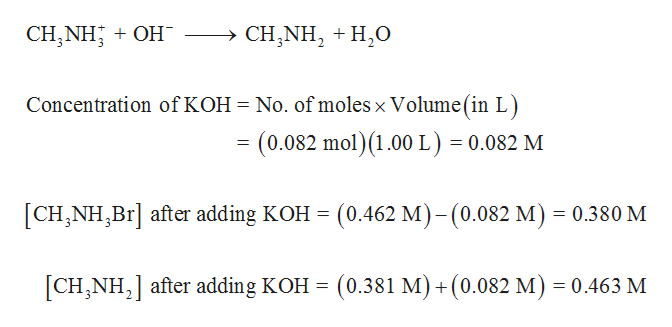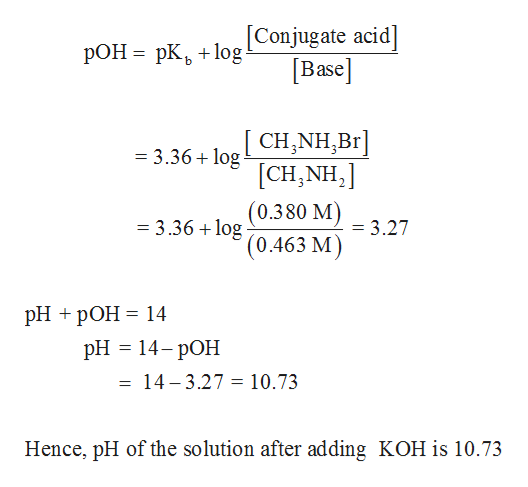# A buffer solution contains 0.462 M CH3NH3Br and 0.381 M CH3NH2 (methylamine). Determine the pH change when 0.082 mol KOH is added to 1.00 L of the buffer.pH after addition − pH before addition = pH change = ______

Question

A buffer solution contains 0.462 M CH3NH3Br and 0.381 M CH3NH2 (methylamine). Determine the pH change when 0.082 mol KOH is added to 1.00 L of the buffer.

pH after addition − pH before addition = pH change = ______

check_circle

Step 1

pKb of CH3NH2 is 3.36.

pOH of the solution can be determined using Henderson-Hasselbalch equation. pH can be determined from the pOH value as follows,help_outlineImage TranscriptioncloseHenderson Hasselbalch expression: [Conjugate acid] Base РОН 3 рК, +1оg [CH,NH,Br [CH,NH2 (0.462 M) ' (0.381 M =3.36 log = 3.36+log = 3.44 PH + pОН %3D 14 pH 3 14-рОН 14-3.44 10.56 Hence, pH of the solution before adding KOH is 10.56 fullscreen
Step 2

On addition of KOH, it reacts with CH3NH3Br forming CH3NH2. Hence, concentration of CH3NH3Br decreases whereas concentration of CH3NH2 increases after the addition of KOH. Hence, the concentration of CH3NH3Br and CH3NH2 is calculated.help_outlineImage TranscriptioncloseCH,NH OH - > CH,NH2H0 Concentration of KOH No. of moles x Volume(in L) (0.082 mol)(1.00 L) = 0.082 M = [CH,NH,Br after adding KOH= (0.462 M)-(0.082 M) 0.380 M CHNH after adding KOH = (0.381 M) +(0.082 M) = 0.463 M fullscreen
Step 3

pH of the solution after adding KOH ...help_outlineImage Transcriptionclose[Conjugate acid] Base РОН - рК, +1оg CH,NH,Br] [CH,NH2 (0.380 M) =3.36+log = 3.36 +log = 3.27 '(0.463 M) PH + pОН 3 14 рH — 14- рОН = 14-3.27 = 10.73 Hence, pH of the solution after adding KOH is 10.73 fullscreen

### Want to see the full answer?

See Solution

#### Want to see this answer and more?

Solutions are written by subject experts who are available 24/7. Questions are typically answered within 1 hour.*

See Solution
*Response times may vary by subject and question.
Tagged in

### Equilibrium Concepts